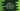# Print the even and odd index characters of a string in pythonIn this post, we will learn how to print the characters at even and odd index of a string in Python. With this program, you will learn how to check if a value is even or odd, how to iterate through a string in python and how to read characters of a string in python.

This program will take one string as input from the user and it will print all the characters at even and odd positions in two different strings.

### What are even and odd values:

A number is called even if it is perfectly divisible by 2. That means, if we are dividing that number by 2, the remainder will be 0. For example, 4 is an even number.

We can use modulo, % operator to check if a number is even or odd in python. If n is the number, n%2 will be always 0 if n is even.

Similarly, a number is called odd if it is not perfectly divisible by 2. If we divide the number by 2, the remainder will be 1 always. For example, 11 is odd.

### Algorithm:

To solve this problem, we can use the below algorithm:

• Create two empty arrays to hold the even and odd index characters.
• Take the string as input from the user.
• Iterate through the characters of the string one by one.
• For each character, check the index is even or odd. Insert that character to even or odd index array.

## Python program:

Below is the complete python program:

``````given_string = input('Enter a string: ')

even_chars = []
odd_chars = []

for i in range(len(given_string)):
if i % 2 == 0:
even_chars.append(given_string[i])
else:
odd_chars.append(given_string[i])

print('Odd characters: {}'.format(odd_chars))
print('Even characters: {}'.format(even_chars))``````

Here,

• given_string is the user input string. We are taking this string as input from the user and storing it in given_string.
• even_chars and odd_chars are arrays to hold the even and odd index characters. We are initialize them as empty arrays.
• The for loop is iterating through the characters of the string one by one. It checks the current index value and appends the character to even_chars or odd_chars based on the current index.
• The last two lines are printing the content of odd_chars and even_chars, i.e. all odd and even characters.

### Sample Output:

If you run this program, it will print output as like below:

``````Enter a string: hello
Odd characters: ['e', 'l']
Even characters: ['h', 'l', 'o']

Enter a string: lazyfox
Odd characters: ['a', 'y', 'o']
Even characters: ['l', 'z', 'f', 'x']``````

You can also add the characters to an empty string instead of using arrays.